output.to from Sideway

Draft for Information Only

# Content

` Acoustic 3D Plane Wave  Continuity Equation, 3D   Euler's Equation of Inviscid Motion, 3D`

## Acoustic 3D Plane Wave

The 1D plane wave can be extended into a 3D plane wave by considering a rectangular volume with coordinates x, y, z.

### Continuity Equation, 3D

For a control volume, from the principle of conservation of mass, the instantaneous rate of change of mass in a control volume equals to the net mass flux flow into or out of the control volume, therefore: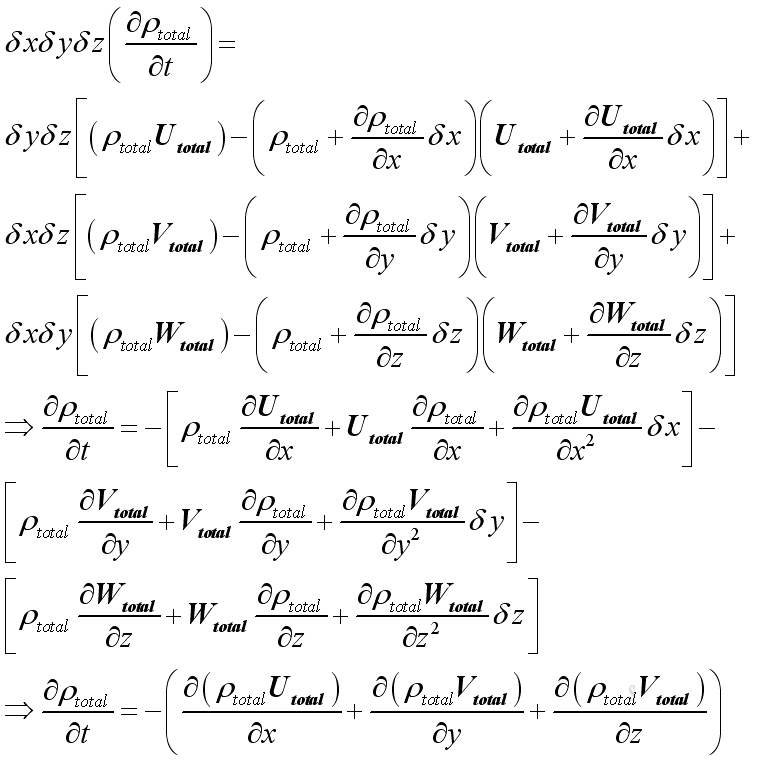As in 1D plane wave, the linearized form is :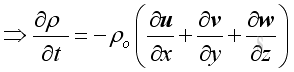And the time derivative of the equation is: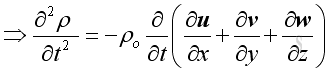### Euler's Equation of Inviscid Motion, 3D

For a control volume, from principle of momentum conservation, the instantaneous rate of change of net momentum of a control volume equals to the net applied force and the net momentum change due to the momentum flux flow into or out of the control volume. The applied force in this case is pressure only and no other forces, no gravity, no viscous force etc., then:

In x direction: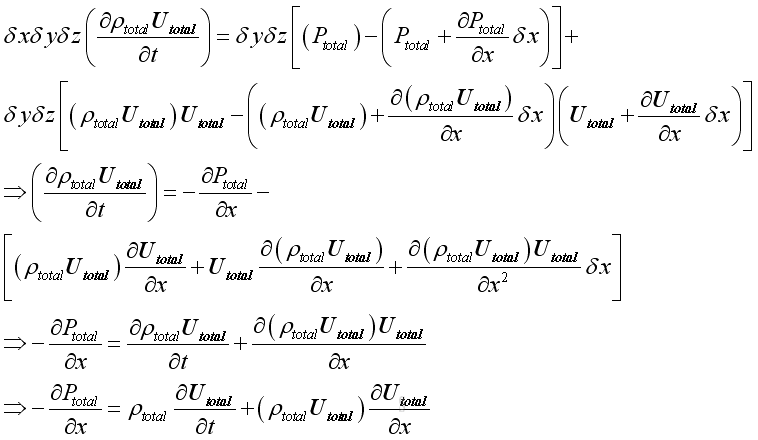As in 1D plane wave, the linearized form is :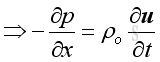And the position derivative of the equation is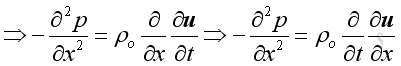Similarly in y and z direction is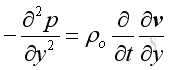and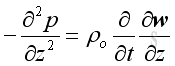And get::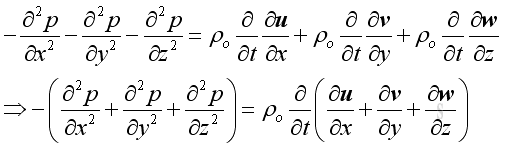Equate two equations::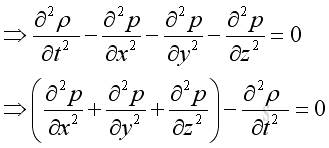Wave Equation, 3D

Applying the equation of state and substitute the speed of propagation, imply.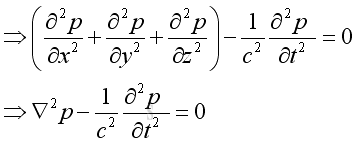Plane Wave in 3D

Assume harmonic sound wave, imply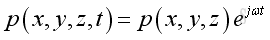substitute the harmonic function into the 3D wave equation and take the time differential operation, imply: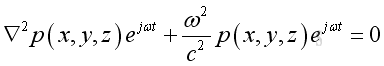and get the Helmholtz equation: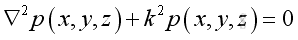Assume the pressure is of format: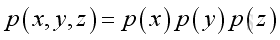substitute the pressure function into the Helmholtz equation, and take the Laplacian differential operation: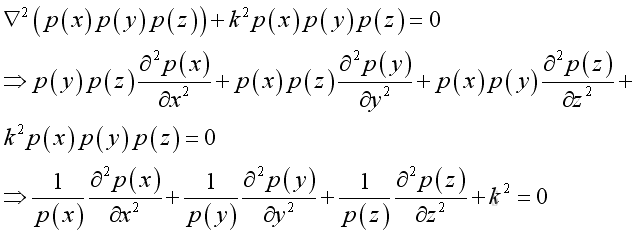let the dispersion equation :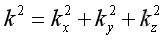substitute into the Helmholtz equation: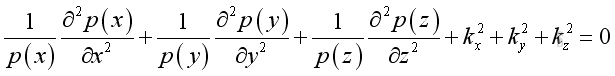By separating the variable, imply: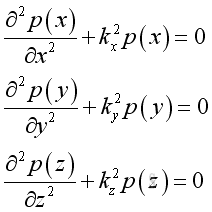The corresponding solutions are: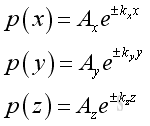substute all into the pressure function and get the solution of wave equation is: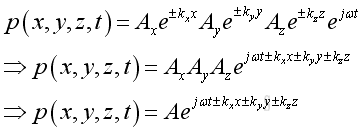Therefore a propagating wave can be decomposed into three components along three axes. A positive sign implies a forward wave from the source to the free space. While a negative sign implies a reflected wave. With the dispersion of the value, k into three components along the three axes

©sidewayReferences

1. Michael P. Norton; Denis G. Karczub,, 2003, Fundamentals of Noise and Vibration Analysis for Engieer, Cambridge, United Kingdom
2. G. Porges, 1977, Applied Acoustics, Edward Arnold Limited, Britain
3. Douglas D. Reynolds, 1981, Engineering Principles of Acoustics:; Noise and Vibration Control, Allyn and Bacon, USA
4. Conrad J. Hemond, 1983, Engineering Acoustics & Noise Control, Prentice-Hall, USA
5. F. Fahy, 2001, Foundations of Engineering Acoustics, Academic Press, UK
6. D.A. Biew; C.H. Hansen, 1996, Engineering Noise Control: Theory and Practice, E & FN Spon, New YorkID: 101000014 Last Updated: 10/16/2010 Revision: 0 Ref:Home (5)

Business

Management

HBR (3)

Information

Recreation

Hobbies (7)

Culture

Chinese (1097)

English (336)

Reference (66)

Computer

Hardware (149)

Software

Application (187)

Digitization (24)

Numeric (19)

Programming

Web (644)CSS (SC)

ASP.NET (SC)

HTML

Knowledge Base

Common Color (SC)

Html 401 Special (SC)

OS (389)MS Windows

Windows10 (SC)

.NET Framework (SC)

DeskTop (7)

Knowledge

Mathematics

Formulas (8)

Number Theory (206)

Algebra (20)

Trigonometry (18)

Geometry (18)

Calculus (67)

Complex Analysis (21)

Engineering

Tables (8)

Mechanical

Mechanics (1)

Rigid Bodies

Statics (92)

Dynamics (37)

Fluid (5)

Control

Acoustics (19)

Biology (1)

Geography (1)

Latest Updated Links

Copyright © 2000-2019 Sideway . All rights reserved Disclaimers last modified on 10 Feb 2019